An ice box used for keeping eatable cold has a total wall area of 1 ${\mathrm{metre}}^{2}$ and a wall thickness of 5.0 cm. The thermal conductivity of the ice box is K = 0.01 joule/metre-s-$°\mathrm{C}$. It is filled with ice at 0$°\mathrm{C}$ along with eatables on a day when the temperature is 30°C. The latent heat of fusion of ice is . The amount of ice melted in one day is ( 1 day = 86,400 seconds )
(a) 776 gms            (b) 7760 gms
(c) 11520 gms         (d) 1552 gms

Concept Questions :-

Conduction
High Yielding Test Series + Question Bank - NEET 2020

Difficulty Level:

Five rods of the same dimensions are arranged as shown in the figure. They have thermal conductivities  and ${\mathrm{K}}_{5}$. When points A and B are maintained at different temperatures, no heat flows through the central rod if :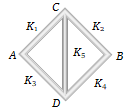(a) ${\mathrm{K}}_{1}={\mathrm{K}}_{4}$ and ${\mathrm{K}}_{2}={\mathrm{K}}_{3}$
(b) ${\mathrm{K}}_{1}{\mathrm{K}}_{4}={\mathrm{K}}_{2}{\mathrm{K}}_{3}$
(c) ${\mathrm{K}}_{1}{\mathrm{K}}_{2}={\mathrm{K}}_{3}{\mathrm{K}}_{4}$
(d) $\frac{{\mathrm{K}}_{1}}{{\mathrm{K}}_{4}}=\frac{{\mathrm{K}}_{2}}{{\mathrm{K}}_{3}}$

Concept Questions :-

Conduction
High Yielding Test Series + Question Bank - NEET 2020

Difficulty Level:

A hot metallic sphere of radius r radiates heat. It's rate of cooling is
(a) Independent of r              (b) Proportional to r
(c) Proportional to ${\mathrm{r}}^{2}$             (d) Proportional to 1/r

Concept Questions :-

Newton's law of cooling
High Yielding Test Series + Question Bank - NEET 2020

Difficulty Level:

A solid copper sphere (density $\mathrm{\rho }$ and specific heat capacity c) of radius r at an initial temperature 200K is suspended inside a chamber whose walls are at almost 0K. The time required (in $\mathrm{\mu }$s) for the temperature of the sphere to drop to 100 K is
(a) $\frac{72}{7}\frac{\mathrm{r\rho c}}{\mathrm{\sigma }}$                  (b) $\frac{7}{72}\frac{\mathrm{r\rho c}}{\mathrm{\sigma }}$
(c) $\frac{27}{7}\frac{\mathrm{r\rho c}}{\mathrm{\sigma }}$                  (d) $\frac{7}{27}\frac{\mathrm{r\rho c}}{\mathrm{\sigma }}$

Concept Questions :-

Newton's law of cooling
High Yielding Test Series + Question Bank - NEET 2020

Difficulty Level:

One end of a copper rod of uniform cross-section and of length 3.1 m is kept in contact with ice and the other end with water at 100°C. At what point along it's length should a temperature of 200°C be maintained so that in steady state, the mass of ice melting be equal to that of the steam produced in the same interval of time. Assume that the whole system is insulated from the surroundings. Latent heat of fusion of ice and vaporisation of water are 80 cal/gm and 540 cal/gm respectively .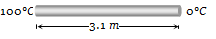(a) 40 cm from 100°C end                    (b) 40 cm from 0°C end
(c) 125 cm from 100°C end                   (d) 125 cm from 0°C end

Concept Questions :-

Conduction
High Yielding Test Series + Question Bank - NEET 2020

Difficulty Level:

A sphere and a cube of same material and same volume are heated upto same temperature and allowed to cool in the same surroundings. The ratio of the amounts of radiations emitted will be
(a) 1 : 1                 (b) $\frac{4\mathrm{\pi }}{3}:1$
(c) ${\left(\frac{\mathrm{\pi }}{6}\right)}^{1/3}:1$          (d) $\frac{1}{2}{\left(\frac{4\mathrm{\pi }}{3}\right)}^{\frac{2}{3}}:1$

Concept Questions :-

Stefan-Boltzmann law
High Yielding Test Series + Question Bank - NEET 2020

Difficulty Level:

The temperature of the two outer surfaces of a composite slab, consisting of two materials having coefficients of thermal conductivity K and 2K and thickness x and 4x, respectively are ${\mathrm{T}}_{2}$ and ${\mathrm{T}}_{1}$ (${\mathrm{T}}_{2}$ > ${\mathrm{T}}_{1}$). The rate of heat transfer through the slab, in a steady state is $\left(\frac{\mathrm{A}\left({\mathrm{T}}_{2}-{\mathrm{T}}_{1}\right)\mathrm{K}}{\mathrm{x}}\right)\mathrm{f}$, with f which equals to -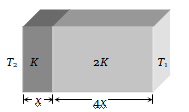(a) 1
(b) $\frac{1}{2}$
(c) $\frac{2}{3}$
(d) $\frac{1}{3}$

Concept Questions :-

Conduction
High Yielding Test Series + Question Bank - NEET 2020

Difficulty Level:

The figure shows a system of two concentric spheres of radii ${\mathrm{r}}_{1}$ and ${\mathrm{r}}_{2}$ and kept at temperatures ${\mathrm{T}}_{1}$ and ${\mathrm{T}}_{2}$, respectively. The radial rate of flow of heat in a substance between the two concentric spheres is proportional to -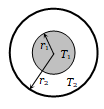(a) $\frac{{\mathrm{r}}_{1}{\mathrm{r}}_{2}}{\left({\mathrm{r}}_{1}-{\mathrm{r}}_{2}\right)}$
(b) $\left({\mathrm{r}}_{1}-{\mathrm{r}}_{2}\right)$
(c) $\left({\mathrm{r}}_{1}-{\mathrm{r}}_{2}\right)\left({\mathrm{r}}_{1}{\mathrm{r}}_{2}\right)$
(d) In $\left(\frac{{\mathrm{r}}_{2}}{{\mathrm{r}}_{1}}\right)$

Concept Questions :-

Conduction
High Yielding Test Series + Question Bank - NEET 2020

Difficulty Level:

The graph shown in the adjacent diagram, represents the variation of temperature (T) of two bodies, x and y having same surface area, with time (t) due to the emission of radiation. Find the correct relation between the emissivity (e) and absorptivity (a) of the two bodies .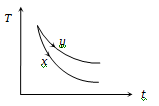(a)
(b)
(c)
(d)

Concept Questions :-

High Yielding Test Series + Question Bank - NEET 2020

Difficulty Level:

The plots of intensity versus wavelength for three black bodies at temperatures ${\mathrm{T}}_{1}$${\mathrm{T}}_{2}$ and ${\mathrm{T}}_{3}$ respectively are as shown. Their temperature are such that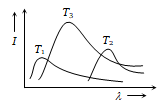(a) ${\mathrm{T}}_{1}$>${\mathrm{T}}_{2}$>${\mathrm{T}}_{3}$
(b)  ${\mathrm{T}}_{1}$>${\mathrm{T}}_{3}$>${\mathrm{T}}_{2}$
(c) ${\mathrm{T}}_{2}$ >${\mathrm{T}}_{3}$ >${\mathrm{T}}_{1}$
(d) ${\mathrm{T}}_{3}$>${\mathrm{T}}_{2}$${\mathrm{T}}_{1}$

Concept Questions :-

Wien displacement law